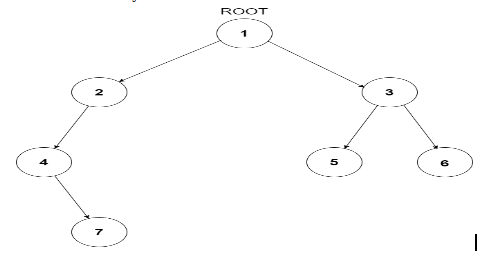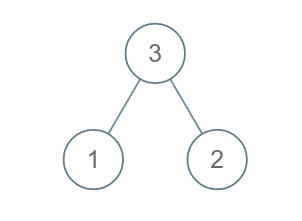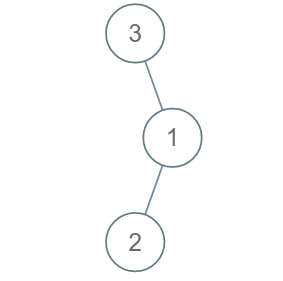Problem title
Difficulty
Avg time to solve

Easy
15 mins
Easy
15 mins
Insertion In A Singly Linked List
Easy
15 mins
Height of Binary Tree
Easy
15 mins
Easy
12 mins
Count Numbers With Equal First and Last Digit
Moderate
15 mins
Count Numbers Containing Given Digit K Times
Moderate
30 mins
Rotate Clockwise
Easy
15 mins
Prime Digit Sum
Hard
45 mins
Ninja And The Clan
Moderate
24 mins

# Height of Binary Tree

Difficulty: EASY
Avg. time to solve
15 min
Success Rate
80%

Problem Statement

#### Input Format :

``````The first line of the input contains a single integer T, representing the number of test cases.

The first line of each test case will contain the values of the tree’s nodes in the level order form ( -1 for NULL node). Refer to the example for further clarification.
``````

#### Example :

``````Consider the binary tree
````````````The input of the tree depicted in the image above will be like:

1
2 3
4 -1 5 6
-1 7 -1 -1 -1 -1
-1 -1

Explanation :
Level 1 :
The root node of the tree is 1

Level 2 :
Left child of 1 = 2
Right child of 1 = 3

Level 3 :
Left child of 2 = 4
Right child of 2 = null (-1)
Left child of 3 = 5
Right child of 3 = 6

Level 4 :
Left child of 4 = null (-1)
Right child of 4 = 7
Left child of 5 = null (-1)
Right child of 5 = null (-1)
Left child of 6 = null (-1)
Right child of 6 = null (-1)

Level 5 :
Left child of 7 = null (-1)
Right child of 7 = null (-1)

The first not-null node (of the previous level) is treated as the parent of the first two nodes of the current level. The second not-null node (of the previous level) is treated as the parent node for the next two nodes of the current level and so on.

The input ends when all nodes at the last level are null (-1).
``````

#### Output Format :

``````For each test case, the print height of the given binary tree.
``````

#### Note :

``````You do not need to print anything. It has already been taken care of. Just implement the given function.
``````

#### Constraints :

``````1 <= T <= 5
1 <= N <= 3000

Time Limit: 1sec
``````
##### Sample Input 1:

2 3 1 2 -1 -1 -1 -1 3 -1 1 2 -1 -1 -1

1 2

##### Explanation for sample input 1:

For the first test case, the given tree is :For each child left or right or node, we have the utmost one node so height is 1.

For the second test case, the given tree isThe root node has only a right node and the right node has only one node which is the left node so total of two nodes are there so the height is 2.Console